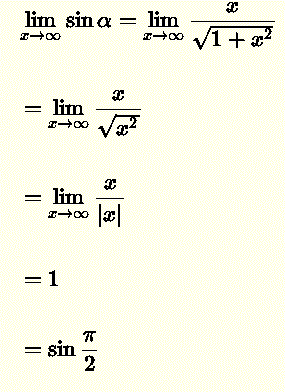# Limit of Arctan(x) as x Approaches Infinity

The lmit of arctan(x) as x approaches infinity is examined using two different approaches. The first one is based on the right triangle and the second is based of the inverse function arctan(x) definition.

## Arctan(x) in a Right Triangle Approach

Let ? = arctan(x) which gives tan ? = x = x / 1 and use the definition of tan x in a right triangle to visualise x = tan ? .

Geometrical Approach
As x increases indefinitely, geometrically ? approaches ?/2 and hence we write the limitAlgebraic Approach
We can also use sin (?) given byCalculate the limitwhich gives $\lim_{x \to \infty} \sin \alpha = \sin \dfrac{\pi}{2}$ Using the limit of composite functions, we write $$\lim_{x \to \infty} \sin \alpha = \sin (\lim_{x \to \infty} \alpha)$$ and the fact that
$$\dfrac{\pi}{2}$$ is a constant hence $$\dfrac{\pi}{2} = \lim_{x \to \infty} \dfrac{\pi}{2}$$, we write $\lim_{x \to \infty} \sin \alpha = \sin (\lim_{x \to \infty} \; \alpha ) = \sin \lim_{x \to \infty} \dfrac{\pi}{2}$ Using $$\alpha = \arctan(x)$$ and the above, we write $\lim_{x \to \infty} \; \alpha = \lim_{x \to \infty} \; \arctan(x) = \dfrac{\pi}{2}$

## Tan (x) and Arctan(x) Graphs Approach

Below is shown the graph of the function function $$y = \tan x$$ (in blue) on the interval $$(-\dfrac{\pi}{2} , \dfrac{\pi}{2})$$, with $$x = \dfrac{\pi}{2}$$ and $$x = - \dfrac{\pi}{2}$$ being vertical asymptotes (broken line blue). It is a one to one function function and therefore has the $$y = \arctan (x)$$ as shown below (in red).
The vertical asymptotes of $$y = \tan x$$ becomes horizontal asymptotes (broken line red) of the inverse function $$y = \arctan (x)$$ which by definition may be written using limits as follows:
$\lim_{x \to \infty} \arctan(x) = \dfrac{\pi}{2}$ and $\lim_{x \to -\infty} \arctan(x) = -\dfrac{\pi}{2}$Fig. 2 - Graph of $$\tan(x)$$ and $$\arctan(x)$$